https://doi.org/10.5194/tc-14-131-2020
https://doi.org/10.5194/tc-14-131-2020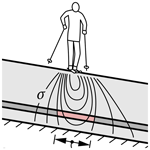# Modeling snow slab avalanches caused by weak-layer failure – Part 2: Coupled mixed-mode criterion for skier-triggered anticracks

Philipp L. Rosendahl and Philipp Weißgraeber
Abstract

Using the analytical model presented in Part 1 of this two-part paper, a new conceptual understanding of anticrack nucleation in weak layers is proposed. To obtain a sufficient condition for onset of failure, two necessary conditions must be satisfied simultaneously: (i) the weak layer must be overloaded in terms of stress and (ii) the initiating crack must release enough energy for the formation of new surfaces. This so-called coupled criterion was proposed by . No assumptions on initial defects within the weak layer are needed. Instead, the failure criterion provides both critical loading and the size of initiating cracks. It only requires the fundamental material properties strength and fracture toughness as inputs. Crack initiation and subsequent propagation are covered by a single criterion containing both a strength-of-materials and a fracture mechanics condition.

Analyses of skier-loaded snowpacks show the impact of slab thickness and slope angle on critical loading and crack initiation length. In the limit cases of very thick slabs and very steep slopes, we obtain natural avalanche release. A discussion of different mixed-mode stress and energy criteria reveals that a wrong choice of mixed-mode hypotheses can yield unphysical results. The effect of material parameters such as density and compliance on weak-layer collapse is illustrated.

The framework presented in this two-part series harnesses the efficiency of closed-form solutions to provide fast and physically sound predictions of critical snowpack loads using a new conceptual understanding of mixed-mode weak-layer failure. It emphasized the importance of both stress and energy in avalanche release.

Share
Dates
1 Introduction

To study the onset of weak-layer failure, fracture mechanics models have been proposed that extend classical stability indices. Classical fracture mechanics is restricted to the analysis of growth of existing cracks. For a crack to propagate, a sufficient energy release is required to overcome the energy barrier for crack growth that originates, e.g., from surface energy and dissipative processes. Fracture mechanics has been applied to the analysis of weak-layer shear cracks and to the propagation of weak-layer collapse . The latter led to the anticrack1 concept for avalanche release . Rendering part of the failure process as a collapse of the weak layer and describing it using fracture mechanics created a new perspective on avalanche release. Here, collapse refers to the sudden loss of volume of the porous weak layer, which can be caused by pure compression or mixed-mode shear and compression loading. The concept provides a physical explanation for remote-triggering and whumpf sounds in avalanche-prone terrain.

In recent years fracture mechanics approaches were used and improved by many researchers . Their use requires an existing crack or flaw within the loaded structure. Hence, in many cases assumptions on the size of such cracks are made (for instance mesoscale “super-weak zones” in weak layers, , or virtual cracks, ). Investigating skier-triggered weak-layer failure, it is often assumed that skier loading causes microscale damage leading to localized flaws . Several studies analyzed the size of such flaws . That is, the initiation of local defects is considered to happen in a separate process and maybe even at a different timescale (McClung1981) than the propagation of the fracture itself. Assuming that skier loading can create uncritical defects implies that several uncritical loading events in temporal sequence can accumulate to a critical size. In other words, such models would predict avalanche release if only enough skiers ski the same slope in close temporal succession, which is an inherent flaw.

In order to characterize the resistance against crack propagation, a new field test, the propagation saw test (PST), was developed. It was first mentioned by and described in detail by . The PST is very similar to the double cantilever beam (DCB) test – an established lab test to quantify fracture mechanics material properties. The latter is standardized , is used by researchers and in industrial applications, and proved to allow for reliable measurements of the fracture toughness .

To link stress-based stability indices and fracture mechanics criteria, combined models were proposed. compare critical crack lengths obtained from a discrete element study to the size of overloaded areas of weak layers to analyze the ability of flaws to initiate and also propagate. The size of the overloaded area is estimated from closed-form analytical solutions for skier-loaded snowpacks . Their parametric study shows realistic parameter dependences and includes the case of self-triggered natural avalanche release without additional load. Although the critical crack length considered by is not a direct fracture mechanics material property, this work is among the first to directly link stability indices (which are based on the strength of materials) and fracture mechanics. Similar efforts were made by , who consider shear failure only, and , who correlate a new stability index based on finite element analyses with a model for critical lengths for mixed-mode crack propagation given by .

Besides mechanical models that aim for closed-form analytical descriptions of the processes within the snowpack, numerical models were developed to study nonlinear failure processes within the snowpack. provide a comprehensive numerical model using a cohesive zone approach for weak-layer fracture. Using this established process zone approach, they study mixed-mode failure criteria and the competition of shear and collapse. use the discrete element method to analyze weak-layer fracture in propagation saw tests. In order to model macroscale crack propagation, the particle size in the simulations was chosen to be significantly larger than microstructural lengths. The results are in good agreement with experimentally obtained critical crack lengths and also with deformations derived from particle tracking velocimetry . The recent model proposed by uses the material point method with a plastic flow rule for hardening that is modified to account for local softening of the weak layer. In their model, the volumetric plastic strain of the porous weak layer controls the energy dissipation of the fracture process. The model recovers the onset of weak-layer failure, the propagation of the failure across a slope and the release and downslope flow of the avalanche . An overview of numerical modeling efforts is given by . Numerical methods are typically more comprehensive; i.e., more competing effects can be studied within the same model. However, due to computational efforts parametric studies are expensive, and general parameter dependencies cannot be derived directly. They can only be derived from observed results of numerical studies and might not apply outside of considered parameter domains.

The present work aims at providing a physical explanation for skier-triggered anticrack nucleation in weak layers. For this purpose we propose a unified failure criterion that directly links strength of materials and fracture mechanics. The criterion accounts for mixed-mode shear and compression loading. We employ closed-form analytical expressions for weak-layer stresses and energy release rates of cracks within the weak layer given in Part 1 of this series .

2 Finite fracture mechanics criterion for skier-triggered anticrack nucleation

Dry-snow slab avalanche release is typically preceded by the nucleation of an anticrack within the weak layer. As discussed by many researchers, skier-triggered weak-layer collapse is a problem of both strength of materials and fracture mechanics (see, e.g., Schweizer et al.2003; Gaume and Reuter2017). In the following, we will show that applying either one or the other exclusively yields contradictory and unphysical predictions in certain situations. This is because infinitesimal crack growth is impossible when no initial crack is present and stresses at existing crack tips are infinite. However, assuming the sudden nucleation of a crack of finite size and combining both strength of materials and fracture mechanics in one unified failure criterion resolves apparent contradictions. Let us illustrate this with the following examples.

## 2.1 Why both strength and toughness matter

Consider the problem of edge crack nucleation in four-point bending tests. Figure 1 shows the results of 299 such experiments on rectangular homogeneous wooden beams reported by . Depicted are maximum local stresses at failure computed from the critical loading at failure. Self-similar specimens of different size but constant length-to-height ratio are tested in this size-effect study. According to the strength of materials, failure occurs when the maximum stress within the beam reaches the material's strength:

$\begin{array}{}\text{(1)}& {\mathit{\sigma }}_{\mathrm{f}}={\mathit{\sigma }}_{\mathrm{c}}.\end{array}$

However, this simple expression is valid only for sufficiently large beams. The experiments in Fig. 1 show that small specimens fail at considerably larger loads than predicted by Eq. (1). The phenomenon is a so-called size effect and is for instance also present at small holes and thin adhesive layers . In structures with localized stress concentrations the initiation of cracks is observed at loads causing stresses that locally exceed the material’s strength. The behavior originates from an insufficient energy release for crack formation.2 We will come back to this problem in the following, showing that modern failure criteria can explain and predict such effects.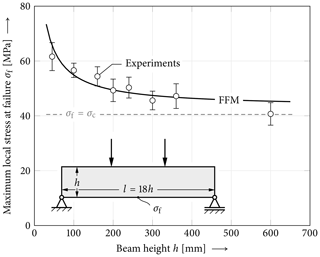Figure 1Size effect of wood beams in 299 self-similar four-point bending experiments. Experimental results reported by are given as mean and standard deviation. The out-of-plane width of the beams is always b=45 mm. The strength-of-materials solution (dashed) and finite fracture mechanics (FFM) predictions (solid) are shown.

As a second example, consider a homogeneous isotropic bar subjected to a critical strain $\stackrel{\mathrm{^}}{\mathit{\epsilon }}$ at which it fails. The critical strain is associated with a critical stress $\stackrel{\mathrm{^}}{\mathit{\sigma }}$. After rupture the broken bar is of course stress- and strain-free. It stores no elastic energy. Hence, the energy released during the fracture process is the entire energy which was stored in the bar prior to fracture:

$\begin{array}{}\text{(2)}& \mathrm{\Delta }\mathrm{\Pi }=-\frac{\mathrm{1}}{\mathrm{2}}Al\stackrel{\mathrm{^}}{\mathit{\sigma }}\stackrel{\mathrm{^}}{\mathit{\epsilon }}=-\frac{\mathrm{1}}{\mathrm{2}}Al\frac{{\stackrel{\mathrm{^}}{\mathit{\sigma }}}^{\mathrm{2}}}{E},\end{array}$

where A is the cross section of the bar, l is its length and E is its Young modulus. If the problem is governed by fracture mechanics, the incremental form of the Griffith criterion, $\stackrel{\mathrm{‾}}{\mathcal{G}}=-\mathrm{\Delta }\mathrm{\Pi }/A={\mathcal{G}}_{\mathrm{c}}$, may be used:

$\begin{array}{}\text{(3)}& \frac{\mathrm{1}}{\mathrm{2}}Al\frac{{\stackrel{\mathrm{^}}{\mathit{\sigma }}}^{\mathrm{2}}}{E}={\mathcal{G}}_{\mathrm{c}}A.\end{array}$

It would be wrong to assume that Eq. (3) allows for computing the critical stress at which the bar will break according to

$\begin{array}{}\text{(4)}& \stackrel{\mathrm{^}}{\mathit{\sigma }}=\sqrt{\mathrm{2}\frac{E{\mathcal{G}}_{\mathrm{c}}}{l}},\end{array}$

if the fracture toughness 𝒢c is a known material constant. Equation (4) implies that the bar will fail at arbitrarily small loads provided it is sufficiently long, which obviously contradicts observations. It draws an incorrect conclusion because the problem is not governed by energy exclusively, but certain stress conditions must be satisfied, too.

In order to resolve the contradictions in the above examples, let us reconsider the four-point bending problem. Instead of examining local processes only, i.e., considering local stresses and expecting infinitesimal crack growth from the edge, let us assume the sudden formation of a finite-sized crack. The concept is known as finite fracture mechanics (FFM) and was proposed by . A finite crack can only nucleate when its energy release is larger than the fracture toughness and when the material's strength is exceeded on the entire potential crack length. This so-called coupled stress and energy failure criterion was proposed by .

Let us now consider such finite cracks in thickness direction on the tensile side of the beam shown in Fig. 1. The energy release rate of these cracks increases with their length. Hence, only sufficiently long finite cracks satisfy the energy condition for a given load, or, in other words, a given short finite crack only releases enough energy at very high loads. Stresses decrease linearly with distance from the edge. Hence, longer finite cracks require larger external loads to still satisfy the stress condition on the entire finite crack length. If the energy condition requires a certain crack length Δa, the normalized crack length Δah is longer for beams of smaller height h. Longer normalized crack lengths require higher loads to satisfy the stress condition. Thus, smaller beams can sustain relatively higher external loads and their apparent flexural strength is larger. FFM predicts the effective flexural strength of all 299 four-point bending experiments shown in Fig. 1 using only two fundamental material properties: the uniaxial tensile strength and the mode I fracture toughness.

The above examples show that (i) fracture processes are governed not by one exclusive but by two conditions simultaneously even if one often hides the other and (ii) strength and toughness are independent fundamental material properties and one cannot be computed from the other.

## 2.2 General coupled stress and energy criterion

The concept of finite fracture mechanics is best understood considering the limitations of classical fracture mechanics. The differential energy release rate3 𝒢(a) of an infinitesimal crack advance is only nonzero when the crack increment extends an existing crack (crack growth). It increases with the length of the existing crack and is zero when there is no initial crack (a=0) . Owing to the vanishing energy release rate of nucleating infinitesimal cracks, it must be assumed that the initial crack does not grow infinitesimally from zero length but forms instantaneously and with finite size. The amount of energy ΔΠ released per finite crack increment Δa is nonzero and known as the incremental energy release rate $\stackrel{\mathrm{‾}}{\mathcal{G}}\left(\mathrm{\Delta }a\right)=-\mathrm{\Delta }\mathrm{\Pi }/\mathrm{\Delta }a$.3 Again, the Griffith criterion must hold, and for a finite crack to nucleate the incremental energy release rate must exceed the fracture toughness:

$\begin{array}{}\text{(5)}& \frac{\stackrel{\mathrm{‾}}{\mathcal{G}}\left(\mathrm{\Delta }a\right)}{{\mathcal{G}}_{\mathrm{c}}}\ge \mathrm{1}.\end{array}$

In the case of mixed-mode loading, a generic mixed-mode energy criterion may be formulated according to

$\begin{array}{}\text{(6)}& g\left({\stackrel{\mathrm{‾}}{\mathcal{G}}}_{\mathrm{I}}\left(\mathrm{\Delta }a\right),\phantom{\rule{0.33em}{0ex}}{\stackrel{\mathrm{‾}}{\mathcal{G}}}_{\mathrm{II}}\left(\mathrm{\Delta }a\right),\phantom{\rule{0.33em}{0ex}}{\mathcal{G}}_{\mathrm{Ic}},\phantom{\rule{0.33em}{0ex}}{\mathcal{G}}_{\mathrm{IIc}}\right)\ge \mathrm{1},\end{array}$

where ${\stackrel{\mathrm{‾}}{\mathcal{G}}}_{\mathrm{I}}$ and ${\stackrel{\mathrm{‾}}{\mathcal{G}}}_{\mathrm{II}}$ denote incremental energy release rates in mode I and II. Because the incremental energy release rate $\stackrel{\mathrm{‾}}{\mathcal{G}}$ increases with increasing size of the finite crack Δa, a certain minimum finite crack length is required to satisfy Eq. (6). Hence, the energy condition represents a lower bound for the finite crack length Δa, which is illustrated in Fig. 2b.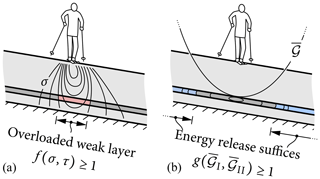Figure 2Coupled stress and energy criterion for the case of a skier-loaded snowpack. On the left the condition of a locally overloaded weak layer and on the right the second condition of sufficient energy release rate are shown. The stress condition has the effect of an upper bound on possible nucleated cracks, whereas the energy condition provides a lower bound on the crack lengths as short cracks do not release sufficient energy.

Besides the critical loading, the size of the initiating finite crack Δa is a second unknown. In order to determine both unknowns, a second necessary condition – a stress condition – is required. A crack can only nucleate when the material is overloaded in terms of stress. In the case of mixed-mode loading the equivalent stress function f must exceed its critical value in every point x on the potential surface Ω of a new finite crack:

$\begin{array}{}\text{(7)}& f\left(\mathit{\sigma }\left(x\right),\mathit{\tau }\left(x\right),\phantom{\rule{0.125em}{0ex}}{\mathit{\sigma }}_{\mathrm{c}},{\mathit{\tau }}_{\mathrm{c}}\right)\ge \mathrm{1}\phantom{\rule{0.25em}{0ex}}\forall \phantom{\rule{0.25em}{0ex}}x\in \mathrm{\Omega }\left(\mathrm{\Delta }a\right).\end{array}$

Cracks typically initiate from stress concentrations. Hence, stresses typically decrease with distance from the point of crack nucleation. At failure the material will only be overloaded in a small region around the point of crack nucleation. Hence, cracks admissible by the stress condition, Eq. (7), can only have a certain maximum length within the overloaded region. The stress criterion constitutes an upper bound for the finite crack length Δa as illustrated in Fig. 2a.

Requiring the simultaneous satisfaction of both the energy and the stress condition,

yields a sufficient condition for crack onset. The concept is known as finite fracture mechanics, and the so-called coupled stress and energy criterion, Eq. (8), provides two equations to determine the two unknowns, critical load and finite crack length. It is physically sound and requires only the fundamental material parameters strength (σc,τc) and toughness (𝒢Ic,𝒢IIc) as input. In particular, no assumptions on initial defects or numerical stabilization or regularization parameters are necessary .

Equation (8) is a unified criterion for crack nucleation and crack growth. Crack initiation is governed by both conditions simultaneously and occurs as a finite crack increment Δa>0. When a crack has formed, stresses at the crack tip are infinite. For perfectly brittle materials the crack increment becomes infinitesimally small Δa→0 when such a crack tip singularity is present. Hence, continuous crack growth according to the Griffith criterion of classical fracture mechanics is recovered.

This general observation also applies to skier-triggered weak-layer collapse. Skier loading induces a stress concentration within the weak layer that may allow for the nucleation of finite-sized anticracks provided the given loading satisfies both the stress and the energy condition. The subsequent stability of this initial anticrack is governed by the energy condition alone.

## 2.3 Mixed-mode strength hypothesis

For the application of the general coupled stress and energy criterion, Eq. (8), to a particular material, both the stress criterion f and the energy criterion g must be chosen accordingly to represent the material behavior . Skier-triggered weak-layer collapse is governed by both shear and compression. A simple and common (for many engineering problems) interaction law determining an effective weak-layer strength under mixed-mode loading is given by the quadratic interaction law

$\begin{array}{}\text{(9)}& {f}_{\mathrm{2}}\left(x\right)=\sqrt{{\left(\frac{\mathit{\sigma }\left(x\right)}{{\mathit{\sigma }}_{\mathrm{c}}^{-}}\right)}^{\mathrm{2}}+{\left(\frac{\mathit{\tau }\left(x\right)}{{\mathit{\tau }}_{\mathrm{c}}}\right)}^{\mathrm{2}}}\end{array}$

between the weak-layer compressive strength ${\mathit{\sigma }}_{\mathrm{c}}^{-}$ and its shear strength τc. In Eq. (9) both strengths, ${\mathit{\sigma }}_{\mathrm{c}}^{-}$ and τc, are assumed constant, and only compressive normal stresses σ(x) are considered.

Failure of shear- and compression-loaded geological materials such as soil and rock is often modeled using the Mohr–Coulomb strength criterion:

$\begin{array}{}\text{(10)}& {f}_{\mathrm{mc}}\left(x\right)=\frac{\mathit{\tau }\left(x\right)}{{\mathit{\tau }}_{\mathrm{c}}^{\mathrm{mc}}\left(\mathit{\sigma }\right)},\end{array}$

where the Mohr–Coulomb shear strength,

$\begin{array}{}\text{(11)}& {\mathit{\tau }}_{\mathrm{c}}^{\mathrm{mc}}\left(\mathit{\sigma }\right)=\left({\mathit{\sigma }}_{\mathrm{c}}^{+}-\mathit{\sigma }\right)\mathrm{tan}\mathit{\varphi },\end{array}$

depends on superimposed normal stresses σ, the tensile strength ${\mathit{\sigma }}_{\mathrm{c}}^{+}$ determined in pure uniaxial tension and the internal friction angle of the material ϕ. Superimposed compression increases sustained shear loading.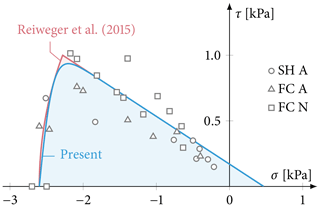Figure 3Smooth (present) and C0-continuous capped Mohr–Coulomb weak-layer failure envelopes with experimental data on weak layers of natural surface hoar (SH N), natural faceted crystals (FC N) and artificial faceted crystals (FC A) as given by , who performed laboratory experiments of weak-layer samples that were loaded in varying mixed-mode conditions. The properties chosen are as given in Fig. 1.

Despite considering the influence of normal stress, the Mohr–Coulomb criterion, Eq. (10), is a pure shear criterion; i.e., it captures only the material's shear failure. Compressive failure is not considered to be the corresponding shear strength, Eq. (11), increases indefinitely with superimposed compression. Modeling porous and collapsible media such as weak layers additionally requires the introduction of a compressive strength. Based on their lab experiments propose to introduce a compressive limit by capping the classical Mohr–Coulomb criterion using

$\begin{array}{}\text{(12)}& {f}_{\mathrm{cap}}\left(x\right)=\frac{\mathit{\tau }\left(x\right)}{{\mathit{\tau }}_{\mathrm{c}}^{\mathrm{cap}}\left(\mathit{\sigma }\right)},\end{array}$

where ${\mathit{\tau }}_{\mathrm{c}}^{\mathrm{cap}}$ is the shear strength in the cap region. It rapidly decreases from its maximum to zero with superimposed compression. In the notation of the present work the equation proposed by reads

$\begin{array}{}\text{(13)}& {\mathit{\tau }}_{\mathrm{c}}^{\mathrm{cap}}\left(\mathit{\sigma }\right)=\mathit{\beta }\sqrt{\mathrm{1}-\frac{{\left(\mathit{\sigma }+{\mathit{\sigma }}_{\mathrm{c}}^{+}\right)}^{\mathrm{2}}}{{\left({\mathit{\sigma }}_{\mathrm{c}}^{-}+{\mathit{\sigma }}_{\mathrm{c}}^{+}\right)}^{\mathrm{2}}}},\end{array}$

with

$\begin{array}{}\text{(14)}& \mathit{\beta }={\mathit{\tau }}_{\mathrm{c}}^{\mathrm{max}}\sqrt{\frac{{\left({\mathit{\sigma }}_{\mathrm{c}}^{+}+{\mathit{\sigma }}_{\mathrm{c}}^{-}\right)}^{\mathrm{2}}}{{\left({\mathit{\sigma }}_{\mathrm{c}}^{+}+{\mathit{\sigma }}_{\mathrm{c}}^{-}\right)}^{\mathrm{2}}-{\left(\frac{{\mathit{\tau }}_{\mathrm{c}}^{\mathrm{max}}}{\mathrm{tan}\mathit{\varphi }}\right)}^{\mathrm{2}}}},\end{array}$

where ${\mathit{\tau }}_{\mathrm{c}}^{\mathrm{max}}$ is the absolute maximum shear strength. It corresponds to the intersection of the cap, Eq. (12), and the Mohr–Coulomb criterion, Eq. (10). Again, ${\mathit{\sigma }}_{\mathrm{c}}^{+}$ and ${\mathit{\sigma }}_{\mathrm{c}}^{-}$ are the tensile and compressive strengths measured in pure uniaxial tension and compression, respectively. Hence, the effective capped Mohr–Coulomb weak-layer shear strength is given as the minimum of the Mohr–Coulomb shear strength ${\mathit{\tau }}_{\mathrm{c}}^{\mathrm{mc}}$ and the cap shear strength ${\mathit{\tau }}_{\mathrm{c}}^{\mathrm{cap}}$:

$\begin{array}{}\text{(15)}& {\mathit{\tau }}_{\mathrm{c}}^{\mathrm{cmc}}\left(\mathit{\sigma }\right)=min\left\{{\mathit{\tau }}_{\mathrm{c}}^{\mathrm{mc}}\left(\mathit{\sigma }\right),\phantom{\rule{0.33em}{0ex}}{\mathit{\tau }}_{\mathrm{c}}^{\mathrm{cap}}\left(\mathit{\sigma }\right)\right\}.\end{array}$

Compressive failure occurs when normal stresses reach the compressive strength $\mathit{\sigma }={\mathit{\sigma }}_{\mathrm{c}}^{-}$. At this point the cap shear strength reduces to zero, ${\mathit{\tau }}_{\mathrm{c}}^{\mathrm{cap}}\left({\mathit{\sigma }}_{\mathrm{c}}^{-}\right)={\mathit{\tau }}_{\mathrm{c}}^{\mathrm{cmc}}\left({\mathit{\sigma }}_{\mathrm{c}}^{-}\right)=\mathrm{0}$, and the capped Mohr–Coulomb criterion

$\begin{array}{}\text{(16)}& {f}_{\mathrm{cmc}}\left(x\right)=\frac{\mathit{\tau }\left(x\right)}{{\mathit{\tau }}_{\mathrm{c}}^{\mathrm{cmc}}\left(\mathit{\sigma }\right)}\end{array}$

is always satisfied (f>1). Likewise tensile failure occurs when $\mathit{\sigma }={\mathit{\sigma }}_{\mathrm{c}}^{+}$ because the Mohr–Coulomb shear strength vanishes, ${\mathit{\tau }}_{\mathrm{c}}^{\mathrm{mc}}\left({\mathit{\sigma }}_{\mathrm{c}}^{+}\right)={\mathit{\tau }}_{\mathrm{c}}^{\mathrm{cmc}}\left({\mathit{\sigma }}_{\mathrm{c}}^{+}\right)=\mathrm{0}$. The capped Mohr–Coulomb criterion proposed by , Eq. (16), is shown in Fig. 3 together with their experimental data on three different weak layers. The criterion provides a proper failure envelope for all investigated weak layers comprising natural surface hoar as well as natural and artificial faceted crystals. However, it kinks at the transition between the classical Mohr–Coulomb criterion and cap, which can cause problems in optimization procedures.

In order to simplify the mathematical formulation of the cap and to provide a smooth envelope, we propose a new effective weak-layer shear strength:

$\begin{array}{}\text{(17)}& {\mathit{\tau }}_{\mathrm{c}}^{\mathrm{scmc}}\left(\mathit{\sigma }\right)=\mathrm{tanh}\left(\mathit{\omega }\left(\mathit{\sigma }-{\mathit{\sigma }}_{\mathrm{c}}^{-}\right)\right){\mathit{\tau }}_{\mathrm{c}}^{\mathrm{mc}}\left(\mathit{\sigma }\right),\end{array}$

where ω controls the sharpness of the transition into the cap region and hence the maximum shear strength. Here we use ω=5. The corresponding stress criterion reads

$\begin{array}{}\text{(18)}& {f}_{\mathrm{scmc}}\left(x\right)=\frac{\mathit{\tau }\left(x\right)}{{\mathit{\tau }}_{\mathrm{c}}^{\mathrm{scmc}}\left(\mathit{\sigma }\right)}.\end{array}$

This criterion is visualized in Fig. 3 alongside the formulation proposed by and the corresponding experimental data obtained from laboratory experiments of samples with weak layers loaded in varied mixed-mode conditions. Both failure envelopes represent the given test data well.

## 2.4 Mixed-mode energy criteria

Mixed-mode energy criteria describe the interaction of crack opening modes I, II and III. Mode I corresponds to crack opening normal to the crack faces, which comprises both tearing and collapse each associated with a distinct fracture toughness, ${\mathcal{G}}_{\mathrm{Ic}}^{+}$ and ${\mathcal{G}}_{\mathrm{Ic}}^{-}$, respectively (see Part 1, Rosendahl and Weißgraeber2020). Modes II and III are shear crack modes and correspond to displacements tangential to the crack faces. The former originates from in-plane shear loading and the latter from out-of-plane shear loading. A crack traveling upslope or downslope is driven by mode I and mode II energy release rates. Cross-slope cracks are mode I and mode III driven. Because PSTs are typically cut upslope, crack propagation is governed by a certain interaction of mode I and II depending on slope angle and slab thickness, and no mode III contributions are present. Both shearing modes II and III are often considered to originate from similar physical mechanisms and associated with similar toughness values 𝒢IIc≈𝒢IIIc.

For the interaction of compressive mode I and mode II loading the following simple general mixed-mode energy criterion,

$\begin{array}{}\text{(19)}& g\left(\mathrm{\Delta }a\right)={\left(\frac{{\mathcal{G}}_{\mathrm{I}}\left(\mathrm{\Delta }a\right)}{{\mathcal{G}}_{\mathrm{Ic}}^{-}}\right)}^{n}+{\left(\frac{{\mathcal{G}}_{\mathrm{II}}\left(\mathrm{\Delta }a\right)}{{\mathcal{G}}_{\mathrm{IIc}}}\right)}^{n},\phantom{\rule{0.25em}{0ex}}n\in \left[\mathrm{1},\mathrm{\infty }\right)\end{array}$

may be used. The exponent n characterizes the strength of the interaction. It is linear for n=1 and vanishes as n→∞, where failure is governed purely by one mode or the other. As a simple and consistent choice we use n=1 throughout the present work. In general, the shape of the strength criterion and the mixed-mode energy criterion are independent.

Other mixed-mode criteria typically used in engineering applications such as the classical criteria by or are not suitable for weak-layer collapse. Both are only applicable when the mode I and mode II toughnesses are of the same order. As discussed in Part 1 , the tearing mode I (${\mathcal{G}}_{\mathrm{Ic}}^{+}$) and shear mode II (𝒢IIc) fracture toughnesses are of the same order of magnitude. However, the collapse mode I fracture toughness ${\mathcal{G}}_{\mathrm{Ic}}^{-}$ is up to 2 orders of magnitude larger than ${\mathcal{G}}_{\mathrm{Ic}}^{+}$. Hence, the above classical criteria are not applicable to weak-layer collapse because of its different micromechanical failure mechanism. The strong anisotropy of the microstructure and the very different failure mechanisms in shear and compression lead to corresponding strength values of a different order of magnitude for shear and compression.

## 2.5 Solution of the coupled criterion

As discussed in Sect. 2.1, neither an exclusive stress criterion nor an exclusive fracture mechanics criterion is sufficient to describe anticrack nucleation. Both are necessary conditions for failure and must be coupled in some way to obtain a sufficient condition for failure. In this work we use the above-discussed mixed-mode criteria for compressive and shear stresses, as well as mode I and II energy release rates, to establish a coupled mixed-mode criterion in the framework of finite fracture mechanics. Using the closed-form analytical solutions for deformations, stresses and energy release rates of a slab on a deformable weak layer presented in Part 1 of this work allows for very efficient evaluations of the individual mixed-mode criteria.

Figure 4 shows the implementation of the coupled stress and energy failure criterion in principle. Initially, the load at failure and the size of initiated crack are unknown. The general normal and shear stress solution of the mechanical model are used to calculate the mixed-mode stress criterion as a function of distance from the skier. Analogously, the solutions for mode I and II energy release rates are used to compute the mixed-mode energy condition as a function of the initially unknown finite crack length. Now the most critical situation satisfying the two conditions simultaneously must be determined. It is associated with the lowest critical extra load. This yields an optimization problem with two free (initially unknown) variables – the critical load and the size of the initiated crack:

The smallest load F satisfying both individual criteria f and g is to be found for any kinematically admissible finite crack Δa. This optimization problem can be treated with standard minimization schemes.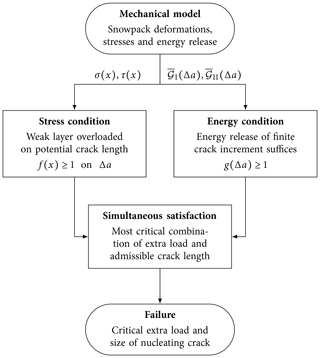Figure 4Solution of the coupled stress and energy criterion for anticrack nucleation. The model presented in the first part of this work provides required stresses and energy release rates. Using the given closed-form analytical solutions, the minimum critical skier force satisfying both conditions can be identified efficiently by employing optimization procedures.

The present implementation of the coupled criterion provided as a Supplement4 requires up to 5 s of computation time on a standard desktop computer. However, the coupled criterion is suited for parallel computing, and the computational cost can be reduced significantly using an iterative solution scheme adapted to the present mixed-mode criteria. This way, the computation time can be reduced to a few milliseconds.

3 Results

In the following, we use the mechanical model derived in Part 1 and the weak-layer failure criterion proposed in Fig. 2 to discuss effects of important snowpack properties on skier-triggered slab avalanche formation. In each study slabs loaded by their own weight and an additional concentrated force are considered. The force represents the outer load that a skier imposes on the snowpack. The given failure criterion predicts the critical magnitude of this additional concentrated force that leads to anticrack nucleation in the weak layer. We call this failure-initiating force the critical skier force.

It is important to point out that the finite crack length Δa and the critical skier force F shown in the following are direct results of the present model and explicitly linked. They cannot be considered or studied individually. The pair of both quantities is a unique solution to the coupled stress and energy criterion, Eq. (8). The critical load is the lowest possible load required to nucleate an initial anticrack of size Δa. The size of the initial crack is different from the critical crack length ac as used, e.g., by . Δa does not represent the critical crack length for crack propagation. It is the size of the initial weak-layer collapse owing to overcritical skier loading. The stability of this crack must be analyzed in a second step.

The second of the two necessary conditions for crack nucleation that are coupled in the coupled criterion (Eq. 8) is the Griffith energy criterion for finite cracks (Eq. 5). That is, when crack nucleation is predicted this condition is always fulfilled. The stability of the initiated crack must be assessed using the Griffith criterion for infinitesimal growth of an existing crack, 𝒢=𝒢c .

The relation between incremental and differential energy release rates is given by

$\begin{array}{}\text{(21)}& \mathcal{G}=\stackrel{\mathrm{‾}}{\mathcal{G}}+a\frac{\partial \stackrel{\mathrm{‾}}{\mathcal{G}}}{\partial a}.\end{array}$

Further, the present situation is a locally positive geometry . That is, the energy release rate increases with crack length in the vicinity of the skier, i.e., $\partial \stackrel{\mathrm{‾}}{\mathcal{G}}/\partial a>\mathrm{0}$. Hence, on account of Eq. (21), 𝒢>𝒢c holds true and the nucleated crack Δa is initially unstable. Of course, the complete analysis of the stability of initiated cracks must also include further aspects such as the touchdown condition of the slab onto the collapsed weak layer, which limits the energy release outside the skier-influenced zone, and must be studied in future work.

We consider the slope angle φ, the thickness of the slab h resting on the weak layer, the slab's density ρ and the slab's Young modulus E.

For static skier loading the local load acting on the snow surface is $F=mgb/{l}_{\mathrm{o}}$, where lo is the effective out-of-plane length of the object, such as the length of skis, and b is the out-of-plane width of the model. In the present work we use b=lo to investigate the total force applied by a skier, which yields F≈0.8 kN for an 80 kg skier using $b={l}_{\mathrm{o}}=\mathrm{1}$ m (Fig. 1). Additionally, different mixed-mode failure criteria and the effect of the slope of the fracture envelope are studied. Each study examines the impact of one individual parameter. All other properties are chosen as given in Fig. 1. If not stated otherwise, the Young modulus of the slab is calculated from density ρ using an empirical power law fit to the data of in plane strain conditions:

$\begin{array}{}\text{(22)}& E=\frac{\mathrm{1}}{\mathrm{1}-{\mathit{\nu }}^{\mathrm{2}}}\phantom{\rule{0.33em}{0ex}}\mathrm{5.07}×{\mathrm{10}}^{\mathrm{3}}{\left(\frac{\mathit{\rho }}{{\mathit{\rho }}_{\mathrm{0}}}\right)}^{\mathrm{5.13}}\phantom{\rule{0.125em}{0ex}}\mathrm{MPa},\end{array}$

with the density of ice ρ0=917kg m−3. Except for the specific analysis of different stress criteria, we employ f2 (Eq. 9) as the stress criterion because of its simplicity.

Table 1Material properties used in parametric studies of the finite fracture mechanics criterion.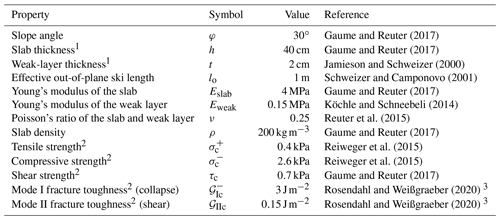1 Thicknesses measured slope normal. 2 Strength and fracture toughness parameters are properties of the weak layer. 3 From data of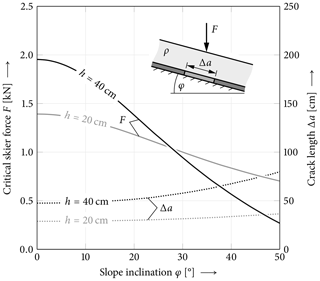Figure 5Effect of the slope angle φ on the critical additional loading F on a defect-free snowpack (solid line) and on the length of predicted initial finite anticrack Δa (dotted line). Steeper slopes cause a significant reduction of the critical skier force and a moderate increase in the crack lengths.

## 3.1 Effects of physical properties

Figure 5 shows the effect of the slope angle φ on the critical extra load F. The critical loading decreases significantly with increasing slope angle. Because of the interaction of compression and shear deformation, and because of the interaction of stress and energy, the change is nonlinear. The length of the finite anticrack Δa initiated at the critical loading increases only moderately with increasing slope angle. The size of the initiated cracks lies between 30 and 80 cm. The thin slab of h=20 cm leads to lower critical loads in flat terrain. However, for steeper slopes this effect is reversed and the critical load of the thicker slab h=40 cm falls below that of the thin slab.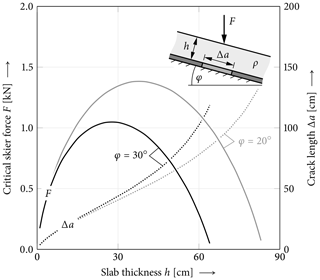Figure 6Critical loading of a snowpack without assumed defects and the size of initiated anticrack as a function of slab thickness. Thicker slabs transfer concentrated loads less localized into the weak layer, allowing for larger point loads. Above a certain thickness, failure is dominated by the slab's own weight, reducing admissible additional loads until self-release occurs. The finite anticrack length increases continuously with increasing slab thickness.

The slab thickness directly affects the critical load. Figure 6 shows a study of this parameter for slab thicknesses up to 90 cm. For low to moderate slab thicknesses the critical extra load sustained by the snowpack increases. On the considered slope angles of 20 and 30 the critical load decreases above 30 and 40 cm slab thickness, respectively. The size of the initial cracks increases monotonously. As observed in Fig. 5, steeper slopes sustain smaller critical loads.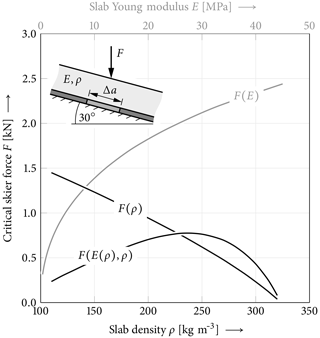Figure 7Effect of slab density ρ on critical skier force F of a defect-free snowpack and the corresponding length of the initiated crack Δa. If the slab's Young modulus is independent of slab density, curve F(ρ), the increasing slab weight reduces the critical skier force. A density-dependent Young modulus according to Eq. (22), curve F(E(ρ),ρ), stiffens denser slabs, which initially dominates failure and increases the critical skier load. Above a certain threshold, the weight gain becomes dominant and the critical skier force reduces distinctly.

Figure 7 shows the impact of both slab density ρ (black lines) and Young's modulus E (gray line). Increasing only the Young modulus stiffens the slab, distributes the skier force more evenly across the weak layer and intuitively increases the critical extra loading. This happens because the Young modulus of the weak layer and the slab are different. Hence, the thickness of the slab controls the bending stiffness of the slab that rests on the foundation of the weak layer. Since the bending stiffness is of a cubic dependence on the slab thickness, this effect is very pronounced. A slab that is stiff in bending will distribute the skier load on a much larger area of the weak layer than in the case of compliant slabs, where the outer load will lead to a pronounced stress concentration just below the point of loading. The influence of the slab density ρ is examined both for a constant Young modulus, $E=\mathrm{const}.$, independent of slab density and for the more realistic case of a density-dependent Young modulus, E=E(ρ), according to Eq. (22). Increasing only the density (at a constant Young modulus) increases the slab weight and hence reduces the critical skier force. If the slab's Young modulus E depends on slab density, denser slabs are stiffer and distribute loads more evenly. As observed for the slab thickness, the initial stiffening allows for larger skier forces. However, at a critical density the slab weight increase dominates failure and critical skier forces drop quickly. In either case, finite anticrack lengths increase with slab density (not shown in Fig. 7). As the critical skier force decreases, the energy release rate at critical loading reduces. This requires longer cracks in order to release sufficient energy for crack formation. For density-dependent Young moduli the stiffening makes this effect even more pronounced.

## 3.2 Comparison of mixed-mode criteria

The coupled stress and energy criterion accounts for shear and compressive failure. In the following the effect of the mixed-mode criteria for stress and energy will be discussed.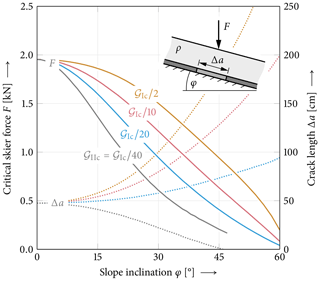Figure 8Comparison of mixed-mode energy criteria for a defect-free snowpack loaded by a point load and the weight of the slab above the weak layer.

The choice of the mixed-mode fracture envelope and the ratio of the mode I and mode II fracture toughness is studied in Fig. 8. The results of the solution of the implicitly coupled criterion are shown for different fracture toughness ratios. For all ratios the critical skier force decreases monotonously with slope angle. With the chosen parameters, natural release is predicted on slopes steeper than φ≈60. For a fracture toughness ratio ${\mathcal{G}}_{\mathrm{IIc}}/{\mathcal{G}}_{\mathrm{Ic}}^{-}>\mathrm{1}/\mathrm{30}$, the corresponding length of the initiated finite cracks increases. When 𝒢IIc is below this threshold the finite crack length decreases.

The effect of the criterion for the interaction of shear and normal stress in the weak layer is studied in Fig. 9. The simple quadratic stress interaction f2, Eq. (9), is compared against the capped Mohr–Coulomb criterion in its smooth formulation fscmc (Eq. 18). The individual constituents of the capped Mohr–Coulomb – the Mohr–Coulomb fmc (Eq. 10) and the capping function fcap (Eq. 12) – are shown as well. The cap fcap corresponds to weak-layer failure due to normal stress only and shows slightly decreasing critical skier forces with increasing slope angle. The Mohr–Coulomb criterion for shear loading of the weak layer fmc shows a strong dependence on the slope angle. The corresponding critical load becomes infinite in flat terrain and vanishes on slopes steeper than 45 for the present set of parameters. The capped Mohr–Coulomb criterion fscmc combines both criteria with a sharp transition at approximately 20. The simple quadratic stress interaction criterion f2 describes a similar trend but provides a smooth transition of the failure load with slope angle. At small slope angles the effect of the slope angle is more pronounced than for the capped Mohr–Coulomb. Yet, for the chosen parameters the critical skier force determined using f2 does not vanish on steep slopes.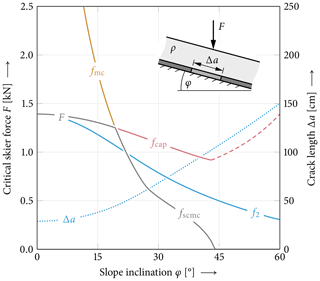Figure 9Comparison of mixed-mode stress criteria for a defect-free snowpack with slab thickness h=20 cm. The quadratic interaction criterion f2, the smooth capped Mohr–Coulomb criterion fscmc, and the constituents of the Mohr–Coulomb criterion fmc and fcap are shown.

4 Discussion of the anticrack nucleation criterion

In the following, results of the parametric studies given above are discussed in order to elucidate basic features of the present failure criterion.

The coupled stress and energy criterion employed in the present work uses linear elastic solutions provided in Part 1 of this work , and the failure condition itself does not account for time-dependence. Hence, any loading which occurs much slower than that of a descending or ascending skier may not be captured correctly. This applies in particular to natural avalanche release due to increased weight of the slab or thermal effects . In these cases, the strain rates are much lower ($<{\mathrm{10}}^{-\mathrm{7}}$s−1) (McClung1979) and the effect of temporal effects like sintering must be accounted for in an extended failure criterion . Since material properties of snow strongly depend on strain rate , they must be chosen to correctly represent the rapid loading by a skier and the corresponding brittle failure behavior.

Local overloading (stress exceeding strength) and sufficient energy release are only necessary conditions of failure . Therefore, we employ the framework of finite fracture mechanics. The concept directly links the two necessary conditions to provide a coupled stress and energy criterion as the sufficient condition for brittle crack onset. Because the length of the initiated crack is a direct result of the solution of the two implicitly coupled equations, it does not have to be assumed. In other words, we do not require the assumption of super-weak zones or initial flaws within the weak layer.

This was also addressed by . They use Föhn's solution to calculate skier-induced shear stress in the weak layer and to determine the length of the overloaded weak layer. This length is then compared to a critical crack length required for crack propagation proposed by to assess the stability of the snowpack. To study layered slabs they use linear elastic finite element analyses to determine the size of the overloaded weak layer. Although employ two criteria, they consider both independently, and their criteria rely on considerably different models and assumptions. The present work derives all input quantities from the same framework and directly couples both conditions. Studying mixed-mode stress and energy criteria (Figs. 8 and 9), we show that mixed-mode weak-layer loading and mixed-mode energy release rate have an important impact on the critical loading of a snowpack.

The present model extends the concept of anticracks by combining strength and energy as coupled conditions for the nucleation of anticracks. Physical interaction criteria of shear and normal stress as well as the mixed-mode energy release are covered. The anticrack model by Heierli extended the understanding of avalanche release and included the remote triggering of avalanches and whumpf sounds for which shear failure models cannot provide physical explanations. However, since the model only considers the weak layer's fracture toughness but not its compliance, important parameter dependences like the effect of slope angles contradict observations . The present model considers the energy release of collapse as well as shear in the condition for initial failure. Collapse is not considered a secondary process as postulated by because normal deformation is directly linked to the initiation of weak-layer failure.

The effect of slope angle φ (Fig. 5) shows a monotonous decrease in the critical load on the snowpack with increasing slope angles. The critical load for φ=0 is finite and corresponds to weak-layer failure in flat terrain causing whumpfs and under certain circumstances remote triggering of avalanches. The critical skier force vanishes in very steep terrain. Here, according to the model no additional external (skier) load can be applied under the given conditions. Natural release is expected for the chosen material properties. The size of initiated cracks increases as the dominant mode I energy release rate reduces, and longer cracks are required to satisfy the energy condition. It should be noted that the size of the initial anticrack is an outcome of the model and does not describe the crack stability directly. In the present case, the crack length becomes long because the mode II energy release rate (shear) is much smaller than the mode I energy release rate (collapse). Comparing the two studied slab thicknesses, several mechanisms interact. Stronger bridging of thicker slabs increases the length of initiating cracks. This causes higher sustained skier forces in flat terrain. The lower strength of the weak layer in shear governs the decrease in the critical loading with slope angle. This finally leads to natural release which occurs of course earlier for thicker and thus heavier slabs.

The effect of slab density ρ and the Young modulus of the slab E are considered in Fig. 7. Increasing the Young modulus of the slab increases bridging and, hence, the capability of distributing the load on a wider area of the weak layer. Considering time-dependent slab stiffening explains why the stability of snowpacks can increase over time. With increasing weight load of the slab owing to increased slab density, the critical load decreases monotonously. If now the Young modulus is assumed to be density-dependent by means of the empirical relation of Scapozza (Eq. 22), these two effects compete. For small slab densities the effect of increasing the Young modulus and accordingly bridging dominates, leading to increasing critical loads. However, for higher slab densities the effect of the extra weight outweighs bridging and the failure load decreases. Eventually natural release is predicted.

The mixed-mode law for the interaction of energy release rates of compressive (mode I) and shear (mode II) deformation of the initiated crack is studied in Fig. 8. Mode mixity is studied by varying the magnitude of the mode II fracture toughness 𝒢IIc relative to a constant mode I fracture toughness 𝒢Ic. For flat terrain the contribution of shear deformation in the weak layer is negligible, and, hence, the parameter variation of 𝒢IIc has no effect on the failure load at φ=0. Part 1 of the present work discusses the fact that the fracture toughness in mode I is significantly higher than that of shear, as the corresponding microstructural failure in compression dissipates much more energy than simple shearing failure of a porous weak-layer structure. Hence, if the mode II fracture toughness is chosen within the order of magnitude of the mode I fracture toughness, a pronounced increase in the length of initiated finite cracks is predicted as the slope angle increases. On steeper slopes failure is dominated by mode II. If the corresponding fracture toughness 𝒢IIc is chosen to be too large, unrealistically long cracks are required to satisfy the energy condition. Crack lengths agree qualitatively with predictions of other models when 𝒢IIc is 20 to 40 times smaller than 𝒢Ic . This ratio of mode I and mode II fracture toughness likely depends on the assumptions of the present work, such as the elastic isotropy of the weak layer and the uncoupled normal and tangential displacements. Further analyses and experimental effort will be required to analyze this aspect in detail.

Figure 9 shows the effect of the given interaction criteria of shear and normal stress in the weak layer. In flat terrain, lateral shear stresses vanish, and using the Mohr–Coulomb criterion only would predict infinite failure loads. Therefore, the capped Mohr–Coulomb was introduced . However, the cap criterion itself shows a peculiar effect when used within the coupled stress and energy criterion. Despite decreasing normal stresses with increasing slope angle, the coupled criterion yields slightly decreased critical skier forces. This occurs because of the increasing mode II energy release rate contribution to the fulfillment of the energy condition. This implicitly allows for shorter initiated cracks and lower failure loads. With a chosen constant shear strength, τc=0.7 kPa, the quadratic interaction criterion f2 does not render the effect of very low shear strengths when low normal loading occurs. Therefore, the decrease in the critical load with increased slope angles is much less pronounced than with the Mohr–Coulomb criterion. This has been addressed by . They pointed out that for steeper slopes the Mohr–Coulomb criterion is the relevant stress criterion and the cap can be neglected. In the present model the transition from the cap criterion to the Mohr–Coulomb criterion occurs near 23. Note that our model considers lateral weak-layer shear stress, i.e., shear stress owing to lateral displacements of the slab only. Transverse shear stress, i.e., shear stress originating from shear deflection of the slab, is not accounted for. The latter is caused by skier loading even in flat terrain and could contribute to lower failure loads predicted by the pure Mohr–Coulomb criterion. However, the former is more relevant for weak-layer failure and dominates in particular on inclined slopes.

The present work studies the onset of weak-layer failure. For a subsequent release of an avalanche, the growth of this crack and the trough-thickness fracture of the slab release are decisive as well. Crack growth is directly controlled by the stability of the initiated and subsequently growing crack. The case of infinitesimal crack growth is covered by Griffith's criterion of linear elastic fracture mechanics (LEFM) and depends only on differential energy release rates in compression and shear. The present model also provides these quantities as an outcome of the model in Part 1, and the stability of the initiated cracks can be assessed in conjunction with the mixed-mode energy criterion (Eq. 19). Once cracks grow long, slab touchdown can occur. This limits the energy release rates of long cracks. Hence, for certain snowpacks no propagation of cracks to a critical size is possible as either the energy release of long cracks is insufficient and the crack stops or the slab itself is too weak and fails close to the initiated weak-layer failure. The stability of cracks under thin slabs and their propagation in flat terrain has been addressed by .

Slab failure is typically induced by a combination of local bending and tension loading of the slab leading to slab fracture . Studies of fracture initiation indicate that the slab fractures in a brittle manner . In the future the present model may be extended to account for fracture of the (layered) slab as a potential consequence of anticrack nucleation and growth.

Since, the propagation of existing cracks is purely energy-controlled (Anderson2017), the crack speed is also only controlled by the energy balance, i.e., in this case the energy balance of energy release at the crack front and the energy required for crack growth. As the latter is just the fracture toughness, measurements of the crack speed could be a way to determine the value of the fracture toughness. However, in inclined terrain, cracks will always expand under mixed-mode conditions. Hence, the evaluation of the crack speed measurements must happen with a model that is able to provide the energy release rates of mode I and mode II.

Effects of the slab and the fracture behavior of the weak layer will always interact, causing a complex failure behavior. Good models may allow for separating effects and, thus, allow for a better understanding of field experiments. For instance, the critical cut length in PST experiments may correlate with slab thickness but does not directly depend on it. Further, and show that edge effects in PSTs can have an important effect. Hence, either field experiments must be modified or models that can account for such edge and size effects need to be established. Similar considerations are necessary for Rutschblock tests. The optimal setup for this skier load stability test must be assessed, and it will determine which properties can be deduced from this test. The recent work by proposes a new way to conduct PSTs. It suggests a change to the geometry of one weak-layer and slab configuration by adding slab thickness. Such an experiment could be of high relevance for identifying the mixed-mode fracture toughness of the particular weak layer.

The present model is based on closed-form equations of the slab and the weak-layer displacement fields. The proposed failure criterion for nucleation of anticrack makes use of this model and solves the implicit equations of the coupled criterion with high efficiency. Since the computational effort is much smaller than for numerical models, the present model can be used readily in large parameter studies or uncertainty quantification analyses.

5 Conclusions

A novel criterion for anticrack nucleation has been proposed on the basis of the closed-form analytical solution proposed in Part 1 of this work.

1. The criterion implicitly links a stress criterion for local overloading of the weak layer with a global fracture mechanics criterion of the energy balance of crack initiation.

2. It is shown that in order to study weak-layer failure the interaction of shear and compression stresses and mixed-mode energy release rates must be considered. Failure is governed by both strength and fracture toughness properties of the weak layer.

3. Parametric studies show that the proposed failure criterion is able to correctly render physical effects observed in slab avalanche release or field tests.

4. The model can be the basis for further analysis of the mixed fracture of the weak layer, the propagation of weak-layer failure and the failure of the slab above the weak layer.

The main limitations of the present model are the assumptions of a homogeneous slab, the isotropy of the weak layer, and the missing coupling of shear and normal displacements. Future works should address these points and investigate the required material properties, in particular elastic properties of the weak layer and the governing fracture and failure envelopes.

Code availability

The analysis code of both the modeling framework in Part 1 and the mixed-mode failure criterion based on this framework is available under https://github.com/2phi/weac (last access: 6 January 2020) (2phi2020).

Author contributions

Both authors defined the scope of the work and developed the present failure model together. PW provided most of the introduction and the discussion. PLR conducted the parametric studies. PLR and PW wrote the final paper with equal contribution.

Competing interests

The authors declare that they have no conflict of interest.

Acknowledgements

We would like to thank Alec van Herwijnen and Johan Gaume for detailed discussions of the present work and the current understanding of the physics of slab avalanche release. We thank Karl Birkeland, Bastian Bergfeld and Ned Bair for the interesting exchange on snow stability and modeling of weak-layer failure. We are grateful for the contributions of the two referees, Michael Zaiser and Jürg Schweizer, and editor Guillaume Chambon, who carefully reviewed the paper.

Financial support

This research has been supported by the German Research Foundation and the Open Access Publishing Fund of Technische Universität Darmstadt.

Review statement

This paper was edited by Guillaume Chambon and reviewed by Jürg Schweizer and Michael Zaiser.

References

2phi: Weak layer anticrack nucleation model, available at: https://github.com/2phi/weac, last access: 6 January 2020. a, b

Anderson, T. L.: Fracture Mechanics, CRC Press, Boca Raton, 4th edn., https://doi.org/10.1201/9781315370293, 2017. a

ASTM Standard D3433-99: Standard Test Method for Fracture Strength in Cleavage of Adhesives in Bonded Metal Joints, ASTM International, West Conshohocken, PA, https://doi.org/10.1520/D3433-99R12, 2012. a

ASTM Standard E399-17: Standard Test Method for Linear-Elastic Plane-Strain Fracture Toughness KIc of Metallic Materials, ASTM International, West Conshohocken, PA, https://doi.org/10.1520/E0399-17, 2017. a

Bader, H. and Salm, B.: On the mechanics of snow slab release, Cold Reg. Sci. Technol., 17, 287–300, 1990. a

Bair, E., Gaume, J., and van Herwijnen, A.: The role of collapse in avalanche release: review and implications for practitioners and future research, in: Proceedings of the International Snow Science Workshop, Breckenridge, CO, USA, 2 October 2016, 24–31, 2016. a

Bair, E. H., Simenhois, R., van Herwijnen, A., and Birkeland, K.: The influence of edge effects on crack propagation in snow stability tests, The Cryosphere, 8, 1407–1418, https://doi.org/10.5194/tc-8-1407-2014, 2014. a

Bažant, Z. P.: Size Effect in Blunt Fracture: Concrete, Rock, Metal, J. Eng. Mech., 110, 518–535, https://doi.org/10.1061/(ASCE)0733-9399(1984)110:4(518), 1984. a

Bažant, Z. P., Zi, G., and McClung, D. M.: Size effect law and fracture mechanics of the triggering of dry snow slab avalanches, J. Geophys. Res.-Sol. Ea., 108, 2119, https://doi.org/10.1029/2002JB001884, 2003. a, b

Benzeggagh, M. L. and Kenane, M.: Measurement of mixed-mode delamination fracture toughness of unidirectional glass/epoxy composites with mixed-mode bending apparatus, Compos. Sci. Technol., 56, 439–449, https://doi.org/10.1016/0266-3538(96)00005-X, 1996. a

Birkeland, K. W., van Herwijnen, A., Reuter, B., and Bergfeld, B.: Temporal changes in the mechanical properties of snow related to crack propagation after loading, Cold Reg. Sci. Technol., 159, 142–152, https://doi.org/10.1016/j.coldregions.2018.11.007, 2019a. a

Birkeland, K. W., van Herwijnen, A., Reuter, B., and Bergfeld, B.: Temporal changes in the mechanical properties of snow related to crack propagation after loading, Cold Reg. Sci. Technol., 159, 142–152, 2019b. a

Bobillier, G., Gaume, J., van Herwijnen, A., Dual, J., and Schweizer, J.: Modeling the propagation saw test with discrete elements, in: Proceedings of the International Snow Science Workshop ISSW 2018, edited by: Fischer, J.-T., Adams, M., Dobesberger, P., Fromm, R., Gobiet, A., Granig, M., Mitterer, C., Nairz, P., Tollinger, C., and Walcher, M., Innsbruck, Austria, 976–980, 2018. a

Broberg, K. B.: Cracks and fracture, Elsevier, 1999. a

Chiaia, B. M., Cornetti, P., and Frigo, B.: Triggering of dry snow slab avalanches: stress versus fracture mechanical approach, Cold Reg. Sci. Technol., 53, 170–178, https://doi.org/10.1016/j.coldregions.2007.08.003, 2008. a

Christen, M., Kowalski, J., and Bartelt, P.: RAMMS: Numerical simulation of dense snow avalanches in three-dimensional terrain, Cold Reg. Sci. Technol., 63, 1–14, 2010. a

Dundurs, J. and Markenscoff, X.: A Green’s function formulation of anticracks and their interaction with load-induced singularities, J. Appl. Mech., 56, 550–555, 1989. a

Felger, J., Rosendahl, P. L., Leguillon, D., and Becker, W.: Predicting crack patterns at bi-material junctions: A coupled stress and energy approach, Int. J. Solids Struct., 164, 191–201, https://doi.org/10.1016/j.ijsolstr.2019.01.015, 2019. a

Fletcher, R. C. and Pollard, D. D.: Anticrack model for pressure solution surfaces, Geology, 9, 419–424, 1981. a

Föhn, P. M. B.: The stability index and various triggering mechanisms, in: Avalanche Formation, Movement and Effects, Proceedings of the Davos Symposium, Davos, September 1986, IAHS Publ., 195–214, 1987. a

Fonselius, M.: Effect of size on the bending strength of laminated veneer lumber, Wood Sci. Technol., 31, 399–413, https://doi.org/10.1007/BF00702562, 1997. a, b

Gaume, J. and Reuter, B.: Assessing snow instability in skier-triggered snow slab avalanches by combining failure initiation and crack propagation, Cold Reg. Sci. Technol., 144, 6–15, https://doi.org/10.1016/j.coldregions.2017.05.011, 2017. a, b, c, d, e, f, g, h, i, j

Gaume, J., van Herwijnen, A., Chambon, G., Birkeland, K. W., and Schweizer, J.: Modeling of crack propagation in weak snowpack layers using the discrete element method, The Cryosphere, 9, 1915–1932, https://doi.org/10.5194/tc-9-1915-2015, 2015. a, b

Gaume, J., van Herwijnen, A., Chambon, G., Wever, N., and Schweizer, J.: Snow fracture in relation to slab avalanche release: critical state for the onset of crack propagation, The Cryosphere, 11, 217–228, https://doi.org/10.5194/tc-11-217-2017, 2017. a, b, c, d, e, f, g

Gaume, J., Gast, T., Teran, J., van Herwijnen, A., and Jiang, C.: Dynamic anticrack propagation in snow, Nat. Commun., 9, 3047, https://doi.org/10.1038/s41467-018-05181-w, 2018. a

Gauthier, D. and Jamieson, B.: Evaluation of a prototype field test for fracture and failure propagation propensity in weak snowpack layers, Cold Reg. Sci. Technol., 51, 87–97, https://doi.org/10.1016/j.coldregions.2007.04.005, 2008a. a

Gauthier, D. and Jamieson, B.: Fracture propagation propensity in relation to snow slab avalanche release: Validating the Propagation Saw Test, Geophys. Res. Lett., 35, L13501, https://doi.org/10.1029/2008GL034245, 2008b. a, b

Hashin, Z.: Finite thermoelastic fracture criterion with application to laminate cracking analysis, J. Mech. Phys. Solids, 44, 1129–1145, https://doi.org/10.1016/0022-5096(95)00080-1, 1996. a

Heierli, J.: Solitary fracture waves in metastable snow stratifications, J. Geophys. Res.-Earth, 110, F02008, https://doi.org/10.1029/2004JF000178, 2005. a

Heierli, J.: Anticrack model for slab avalanche release, PhD thesis, Universität Karlsruhe, 2008. a

Heierli, J. and Zaiser, M.: Failure initiation in snow stratifications containing weak layers: Nucleation of whumpfs and slab avalanches, Cold Reg. Sci. Technol., 52, 385–400, https://doi.org/10.1016/j.coldregions.2007.02.007, 2008. a, b

Heierli, J., Gumbsch, P., and Zaiser, M.: Anticrack nucleation as triggering mechanism for snow slab avalanches, Science, 321, 240–243, https://doi.org/10.1126/science.1153948, 2008. a

Hutchinson, J. W. and Suo, Z.: Mixed Mode Cracking in Layered Materials, J. Appl. Mech., 27, 63–191, https://doi.org/10.1016/S0065-2156(08)70164-9, 1991. a

Jamieson, B. and Johnston, C. D.: Snowpack factors associated with strength changes of buried surface hoar layers, Cold Reg. Sci. Technol., 30, 19–34, https://doi.org/10.1016/S0165-232X(99)00026-9, 1999. a

Jamieson, B. and Schweizer, J.: Texture and strength changes of buried surface-hoar layers with implications for dry snow-slab avalanche release, J. Glaciol., 46, 151–160, https://doi.org/10.3189/172756500781833278, 2000. a

Köchle, B. and Schneebeli, M.: Three-dimensional microstructure and numerical calculation of elastic properties of alpine snow with a focus on weak layers, J. Glaciol., 60, 705–713, https://doi.org/10.3189/2014JoG13J220, 2014. a

Leguillon, D.: Strength or toughness? A criterion for crack onset at a notch, Eur. J. Mech. A-Solid, 21, 61–72, https://doi.org/10.1016/S0997-7538(01)01184-6, 2002. a, b, c

Leguillon, D., Martin, É., and Lafarie-Frenot, M.-C.: Flexural vs. tensile strength in brittle materials, CR Mecanique, 343, 275–281, https://doi.org/10.1016/j.crme.2015.02.003, 2015. a, b

Louchet, F.: A transition in dry-snow slab avalanche triggering modes, Ann. Glaciol., 32, 285–289, 2001. a

Mahajan, P., Kalakuntla, R., and Chandel, C.: Numerical simulation of failure in a layered thin snowpack under skier load, Ann. Glaciol., 51, 169–175, 2010. a

McClung, D. M.: Shear Fracture Precipated by Strain Softening as a Mechanism of Dry Slab Avalanche Release, J. Geophys. Res., 84, 3519–3526, 1979. a, b

McClung, D. M.: Fracture mechanical models of dry slab avalanche release, J. Geophys. Res.-Sol. Ea., 86, 10783–10790, 1981. a

McClung, D. M. and Schweizer, J.: Skier triggering, snow temperatures and the stability index for dry-slab avalanche initiation, J. Glaciol., 45, 190–200, https://doi.org/10.3189/002214399793377121, 1999. a

Monti, F., Gaume, J., van Herwijnen, A., and Schweizer, J.: Snow instability evaluation: calculating the skier-induced stress in a multi-layered snowpack, Nat. Hazards Earth Syst. Sci., 16, 775–788, https://doi.org/10.5194/nhess-16-775-2016, 2016. a

Narita, H.: An experimental study on tensile fracture of snow, Contributions from the institute of Low Temperature Science, 32, 1–37, 1984. a

Podolskiy, E., Chambon, G., Naaim, M., and Gaume, J.: A review of finite-element modelling in snow mechanics, J. Glaciol., 59, 1189–1201, 2013. a

Reiweger, I. and Schweizer, J.: Failure of a layer of buried surface hoar, Geophys. Res. Lett., 37, L24501, https://doi.org/10.1029/2010GL045433, 2010. a

Reiweger, I., Gaume, J., and Schweizer, J.: A new mixed-mode failure criterion for weak snowpack layers, Geophys. Res. Lett., 42, 1427–1432, https://doi.org/10.1002/2014GL062780, 2015. a, b, c, d, e, f, g, h, i, j, k

Reuter, B. and Schweizer, J.: Describing Snow Instability by Failure Initiation, Crack Propagation, and Slab Tensile Support, Geophys. Res. Lett., 45, 7019–7027, https://doi.org/10.1029/2018GL078069, 2018. a

Reuter, B., Schweizer, J., and van Herwijnen, A.: A process-based approach to estimate point snow instability, The Cryosphere, 9, 837–847, https://doi.org/10.5194/tc-9-837-2015, 2015. a, b

Reuter, B., Calonne, N., and Adams, E.: Shear failure of weak snow layers in the first hours after burial, The Cryosphere Discuss., https://doi.org/10.5194/tc-2018-268, 2019. a

Rosendahl, P. L. and Weißgraeber, P.: Modeling snow slab avalanches caused by weak-layer failure – Part 1: Slabs on compliant and collapsible weak layers, The Cryosphere, 14, 115–130, https://doi.org/10.5194/tc-14-115-2020, 2020. a, b, c, d, e, f, g, h, i

Rosendahl, P. L., Weißgraeber, P., Stein, N., and Becker, W.: Asymmetric crack onset at open-holes under tensile and in-plane bending loading, Int. J. Solids Struct., 113-114, 10–23, https://doi.org/10.1016/j.ijsolstr.2016.09.011, 2017. a

Rosendahl, P., Staudt, Y., Schneider, A., Schneider, J., and Becker, W.: Nonlinear elastic finite fracture mechanics: modeling mixed-mode crack nucleation in structural glazing silicone sealants, Mater. Design, 108057, https://doi.org/10.1016/j.matdes.2019.108057, 2019a. a

Rosendahl, P. L., Drass, M., Felger, J., Schneider, J., and Becker, W.: Equivalent strain failure criterion for multiaxially loaded incompressible hyperelastic elastomers, Int. J. Solids Struct., 166, 32–46, https://doi.org/10.1016/j.ijsolstr.2019.01.030, 2019b. a

Rosendahl, P. L., Staudt, Y., Odenbreit, C., Schneider, J., and Becker, W.: Measuring mode I fracture properties of thick-layered structural silicone sealants, Int. J. Adhes. Adhes., 91, 64–71, https://doi.org/10.1016/j.ijadhadh.2019.02.012, 2019c. a

Sapora, A. and Cornetti, P.: Crack onset and propagation stability from a circular hole under biaxial loading, Int. J. Fracture, 214, 97–104, 2018. a

Savage, S. B. and Hutter, K.: The motion of a finite mass of granular material down a rough incline, J. Fluid Mech., 199, 177–215, 1989. a

Scapozza, C.: Entwicklung eines dichte- und temperaturabhängigen Stoffgesetzes zur Beschreibung des visko-elastischen Verhaltens von Schnee, PhD thesis, ETH Zürich, https://doi.org/10.3929/ethz-a-004680249, 2004.  a

Schweizer, J.: Review of dry snow slab avalanche release, Cold Reg. Sci. Technol., 30, 43–57, https://doi.org/10.1016/S0165-232X(99)00025-7, 1999. a, b

Schweizer, J. and Camponovo, C.: The skier's zone of influence in triggering slab avalanches, Ann. Glaciol., 32, 314–320, https://doi.org/10.3189/172756401781819300, 2001. a

Schweizer, J., Jamieson, B., and Schneebeli, M.: Snow avalanche formation, Rev. Geophys., 41, 1016, https://doi.org/10.1029/2002RG000123, 2003. a, b

Sigrist, C. and Schweizer, J.: Critical energy release rates of weak snowpack layers determined in field experiments, Geophys. Res. Lett., 34, L03502, https://doi.org/10.1029/2006GL028576, 2007. a

Stein, N., Weißgraeber, P., and Becker, W.: A model for brittle failure in adhesive lap joints of arbitrary joint configuration, Compos. Struct., 133, 707–718, https://doi.org/10.1016/j.compstruct.2015.07.100, 2015. a

van Herwijnen, A. and Jamieson, B.: High-speed photography of fractures in weak snowpack layers, Cold Reg. Sci. Technol., 43, 71–82, https://doi.org/10.1016/j.coldregions.2005.05.005, 2005. a

van Herwijnen, A. and Jamieson, B.: Snowpack properties associated with fracture initiation and propagation resulting in skier-triggered dry snow slab avalanches, Cold Reg. Sci. Technol., 50, 13–22, https://doi.org/10.1016/j.coldregions.2007.02.004, 2007. a

van Herwijnen, A. and Miller, D. A.: Experimental and numerical investigation of the sintering rate of snow, J. Glaciol., 59, 269–274, https://doi.org/10.3189/2013JoG12J094, 2013. a

van Herwijnen, A., Gaume, J., Bair, E. H., Reuter, B., Birkeland, K. W., and Schweizer, J.: Estimating the effective elastic modulus and specific fracture energy of snowpack layers from field experiments, J. Glaciol., 62, 997–1007, https://doi.org/10.1017/jog.2016.90, 2016. a

Waddoups, M. E., Eisenmann, J. R., and Kaminski, B. E.: Macroscopic fracture mechanics of advanced composite materials, J. Compos. Mater., 5, 446–454, 1971. a

Weißgraeber, P., Felger, J., Geipel, D., and Becker, W.: Cracks at elliptical holes: Stress intensity factor and Finite Fracture Mechanics solution, Eur. J. Mech. A-Solid, 55, 192–198, https://doi.org/10.1016/j.euromechsol.2015.09.002, 2015. a

Weißgraeber, P., Hell, S., and Becker, W.: Crack nucleation in negative geometries, Eng. Fract. Mech., 168, 93–104, https://doi.org/10.1016/j.engfracmech.2016.02.045, 2016a. a, b

Weißgraeber, P., Leguillon, D., and Becker, W.: A review of Finite Fracture Mechanics: crack initiation at singular and non-singular stress raisers, Arch. Appl. Mech., 86, 375–401, https://doi.org/10.1007/s00419-015-1091-7, 2016b. a, b

Here, anticrack refers to the extension of a collapse . This allows for the direct use of the concepts of classical fracture mechanics with the only difference being the sign of the crack tip displacement field. The local displacement field exhibits the same behavior in compression as in tension. It is proportional to the square root of the distance to the crack tip. This should not be confused with the case of a rigid line inclusion, which is also termed “anticrack”, that coincidentally shows the same stress singularity .

In addition, statistical size effects may play a role as well. As shown by , size effects are often a combination of energetic and statistical effects.

See Sect. 2.5 in Part 1 for a comprehensive definition of fracture mechanical quantities.

https://github.com/2phi/weac (last access: 6 January 2020) (2phi2020)